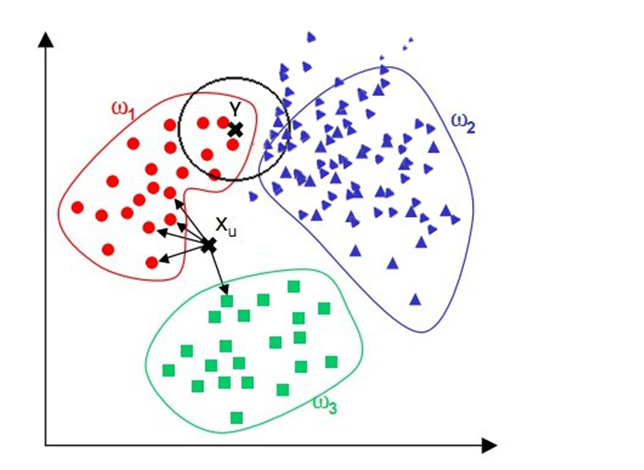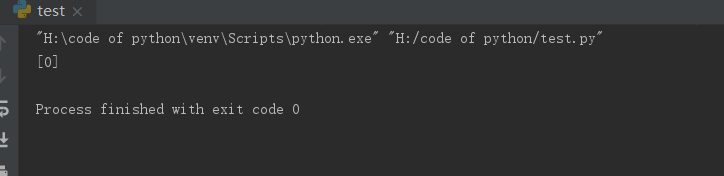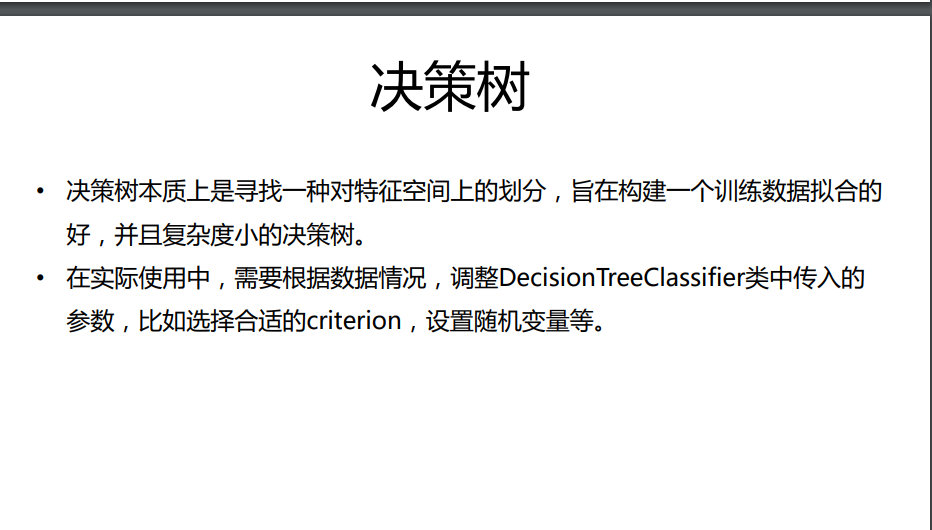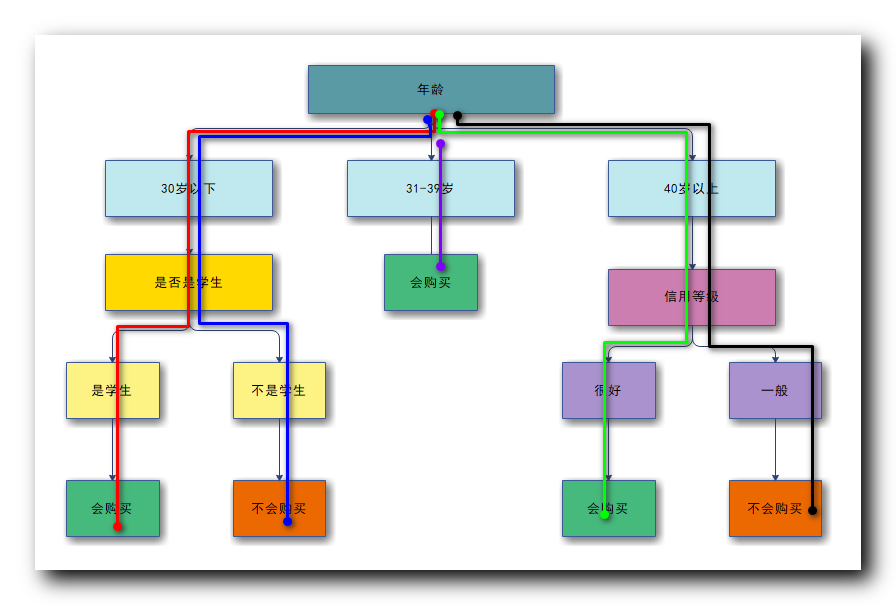• 分类决策规则
万次阅读
2017-09-05 14:56:00

KNN中的K值选取对分类的结果影响至关重要，K值选取的太小，模型太复杂。K值选取的太大，导致分类模糊。那么K值到底怎么选取呢？有人用Cross Validation，有人用贝叶斯，还有的用bootstrap。

而距离度量又是另外一个问题，比较常用的是选用欧式距离。可是这个距离真的具有普适性吗？《模式分类》中指出欧式距离对平移是敏感的，这点严重影响了判定的结果。在此必须选用一个对已知的变换（比如平移、旋转、尺度变换等）不敏感的距离度量。书中提出了采用切空间距离（tangent distance）来替代传统的欧氏距离。

更多相关内容
• kkk近邻法会涉及到三个问题（三要素）：距离度量、kkk值的选择、分类决策规则，下面我们分别介绍。 距离度量 特征空间的两个实例点的距离度量是两个实例点相似程度的反映。距离小，那么相似度大；距离大，那么...

### 回顾

k-近邻算法

前面，我们介绍了 k k -近邻算法，其工作机制就是给定测试样本，基于某种距离度量找出训练集中与其最靠近的k个训练样本，然后基于这k个“邻居”的信息来进行预测。

k k 近邻法会涉及到三个问题（三要素）：距离度量、 k k 值的选择、分类决策规则，下面我们分别介绍。

### 距离度量

特征空间的两个实例点的距离度量是两个实例点相似程度的反映。距离小，那么相似度大；距离大，那么相似度小。 k k -近邻模型的特征空间一般是 n n 维实数向量空间 R n R^n 。使用的距离是欧式距离，但也可以是其他距离，如更一般的 L p L_p 距离（ L p L_p distance）或 M i n k o w s k i Minkowski 距离。

问题定义：设特征空间 X \mathcal{X} n n 维实数向量空间 R n R^n x i , x j ∈ X , x i = ( x i ( 1 ) , x i ( 2 ) , . . . , x i ( n ) ) T , x j = ( x j ( 1 ) , x j ( 2 ) , . . . , x j ( n ) ) T , x i , x j 的 L p 距 离 定 义 为 ： x_i,x_j \in \mathcal{X},x_i = (x_i^{(1)},x_i^{(2)},...,x_i^{(n)})^T,x_j= (x_j^{(1)},x_j^{(2)},...,x_j^{(n)})^T,x_i,x_j的L_p距离定义为：

L p ( x i , x j ) = ( ∑ l = 1 n ∣ x i ( l ) − x j ( l ) ∣ p ) 1 p L_p(x_i,x_j) = (\sum_{l=1}^{n}|x_i^{(l)}-x_j^{(l)}|^p)^{\frac{1}{p}}

这里 p ⩾ 1 p \geqslant 1 ;

1、欧几里得距离（欧式距离 Eucledian Distance）

欧氏距离是最常用的距离计算公式，衡量的是多维空间中各个点之间的绝对距离，当数据很稠密并且连续时，这是一种很好的计算方式。

p = 2 p = 2 时，就是欧式距离，即：

L 2 ( x i , x j ) = ( ∑ l = 1 n ∣ x i ( l ) − x j ( l ) ∣ p ) 1 2 L_2(x_i,x_j) = (\sum_{l=1}^{n}|x_i^{(l)}-x_j^{(l)}|^p)^{\frac{1}{2}}

![这里写图片描述](https://img-blog.csdn.net/20180724082618607?watermark/2/text/aHR0cHM6Ly9ibG9nLmNzZG4ubmV0L0RheWN5bQ==/font/5a6L5L2T/fontsize/400/fill/I0JBQkFCMA==/dissolve/70)

代码：

from math import *
# python3
# 欧式距离
def eculidean_dis(x, y):
return sqrt(sum(pow(a - b, 2) for a, b in zip(x, y)))

x = [1, 3, 2, 4]
y = [2, 5, 3, 1]

print(eculidean_dis(x, y))   # 结果为3.872983346207417


2、曼哈顿距离（Manhattan Distance）

p = 1 p=1 时，称为曼哈顿距离，即：

L 1 ( x i , x j ) = ∑ l = 1 n ∣ x i ( l ) − x j ( l ) ∣ L_1(x_i,x_j) = \sum_{l=1}^{n}|x_i^{(l)}-x_j^{(l)}|

![这里写图片描述](https://img-blog.csdn.net/20180724083940686?watermark/2/text/aHR0cHM6Ly9ibG9nLmNzZG4ubmV0L0RheWN5bQ==/font/5a6L5L2T/fontsize/400/fill/I0JBQkFCMA==/dissolve/70)

代码：

# 曼哈顿距离
def manhattan_dis(x, y):
return sum(abs(a - b) for a, b in zip(x, y))

print(manhattan_dis(x, y))  # 结果为7


3、明可夫斯基距离（Minkowski distance）

明氏距离是欧氏距离的推广，是对多个距离度量公式的概括性的表述：

d i s t ( x i , x j ) = ( ∑ l = 1 n ∣ x i ( l ) − x j ( l ) ∣ p ) 1 p dist(x_i,x_j) = (\sum_{l=1}^{n}|x_i^{(l)}-x_j^{(l)}|^p)^{\frac{1}{p}}

从公式我们可以看出，

• 当p=1,“明可夫斯基距离”变成“曼哈顿距离”
• 当p=2,“明可夫斯基距离”变成“欧几里得距离”
• 当p=∞,“明可夫斯基距离”变成“切比雪夫距离”
![这里写图片描述](https://img-blog.csdn.net/20180724084739707?watermark/2/text/aHR0cHM6Ly9ibG9nLmNzZG4ubmV0L0RheWN5bQ==/font/5a6L5L2T/fontsize/400/fill/I0JBQkFCMA==/dissolve/70)

代码：

# 明可夫斯基距离
def minkowski_dis(x, y, p):
sumval = sum(pow(abs(a - b), p) for a, b in zip(x, y))
mi = 1 / float(p)
return round(sumval ** mi, 3)

print(minkowski_dis(x, y, 3))  # 结果为3.332


### k k 值的选择

k k 值的选择会对 k k 近邻法的结果产生重大影响。

如果 k k 值选择较小，就相当于用较小的领域中的训练实例进行预测，“学习”的近似误差会减小，只有与输入实例较近的训练实例才会对预测起作用，但确定是估计误差回增大。预测结果会对近邻的实例点非常敏感，如果近邻的实例点恰巧是噪声，预测就会出错。

相反如果 k k 值选择较大，就相当于用较大的领域中的训练实例进行预测，近似误差会增大，但估计误差会减小。

特例，如果 k = N k=N ，那么无论输入什么实例，都会简单的预测为训练实例中做多的类，这是的模型就没有意义了，丢失了训练实例中的大量有用信息。

在应用中，我们一般取一个较小的 k k 值，通常采用交叉验证法来选取最优的 k k 值。

### 分类决策规则

k k 近邻法中的分类决策规则往往是多数表决，即由输入实例的 k k 个近邻的训练实例中的多数类决定输入实例的类别。

### 补充拓展

余弦相似度（Cosine Similarity）

余弦相似度用向量空间中两个向量夹角的余弦值作为衡量两个个体间差异的大小。相比距离度量，余弦相似度更加注重两个向量在方向上的差异，而非距离或长度上。

![这里写图片描述](https://img-blog.csdn.net/20180724091440828?watermark/2/text/aHR0cHM6Ly9ibG9nLmNzZG4ubmV0L0RheWN5bQ==/font/5a6L5L2T/fontsize/400/fill/I0JBQkFCMA==/dissolve/70)

代码：

# 余弦相似度
def cosine_dis(x, y):
num = sum(map(float, x * y))
denom = np.linalg.norm(x) * np.linalg.norm(y)
return round(num / float(denom), 3)

x = np.array([3, 4, 1, 5])
y = np.array([3, 4, 1, 5])

print(cosine_dis(x, y))  # 结果为1，这边是相同的两个向量


注：
余弦值的范围在[-1,1]之间，值越趋近于1，代表两个向量的方向越接近；越趋近于-1，他们的方向越相反；接近于0，表示两个向量近乎于正交。

展开全文机器学习
• 类一致性来度量属性的重要性, 逐步加入重要的属性, 当选择的属性子集能够正确分类时, 则获取到决策规则. 算法 中设计了一个规则约简过程, 用来简化所获得的规则, 增强规则的泛化能力. 实验结果表明, 所提出的...
• C4.5算法是机器学习算法中的一种分类决策树算法,其核心算法是ID3算法. 分类决策树算法是从大量事例中进行提取分类规则的自上而下的决策树.
• 基于envi的决策分类方法，在envi classic中创建决策规则模块。
• 一、最临近规则分类（KNN） KNN：通过计算分类数据点，与已有数据集中的所有数据点的距离。取距离最小的前K个点，根据“少数如从多数”的原则，将这个数据点划分为出现次数最多的哪个类别。 如图： 上代码：（一个...

### 如图：上代码：（一个超级简单的例子）

from sklearn.neighbors import KNeighborsClassifier
#创建数据集x 和标签y
x = [,,,]
y = [0,0,1,1]
#设置 K
neigh = KNeighborsClassifier(n_neighbors=3)
#训练
neigh.fit(x,y)
#调用predict()函数，对未知样本进行分类，标签作为输出
print(neigh.predict([[1.1]]))

### 运行截图如下：### 使用伪代码来表示一下就是：

if()
{
if()
{
if()
{

}
}
}


### 意思就是通过多种条件判断达到分类的目的### 参考视频：（中国大学MOOC）https://www.icourse163.org/learn/BIT-1001872001#/learn/content?type=detail&id=1002862574&cid=1003256737

展开全文• 在序决策信息系统中, 定义区间为支配一个特定的对象同时又被另一个特定的对象所支配的所有对象的集合.... 该方法比初始的优势关系粗糙集方法适应性更强, 且所得区间决策规则可直接应用于序信息系统的分类问题.</p>
• 基于决策树的民航气象报文分类统计规则的研究与应用，曹闪，张仁波，民航气象数据库系统自2004年投入运行以来，对业务运行情况没有定量把握，对系统输入与输出资料没有准确的统计报表。通过整体把控��
• 它是一种典型的分类方法，首先对数据进行处理，利用归纳算法生成可读的规则决策树，然后使用决策对新数据进行分析。 本质上决策树是通过一系列规则对数据进行分类的过程。 决策树学习使用决策树（作为预测模型）...
• esri 中国出品 基于ENVI软件的图像分类技术教程之一 基于CART获取规则决策分类，CART算法考虑到每个节点都有成为叶子节点的可能，对每个节点都分配类别。分配类别的方法可以用当前节点中出现最多的类别，也可以...
• 基于本体的判定树分类算法抽取应急决策规则的研究.pdf
• 研究粗糙集理论中可辨识矩阵,扩展了类别特征矩阵,提出一种基于粗糙集理论的最简决策规则算法.该算法根据决策属性将原始决策表分成若干个等价子决策表,借助核属性和属性频率函数对各类别特征矩阵挖掘出最简决策规则....
• 数据挖掘实验 实验指导书 决策树模型 线性回归模型 关联规则 贝叶斯分类 共4个实验.pdf
• 为了将传统的决策树无法管理的、由各种分类算法所发现的大量的有意义的规则进行有效的存储、剪裁和使用,提出了广义决策树结构。它将传统决策树的结构进行扩展,能够以较少的存储代价管理所发现的所有分类规则,且易于...
• 针对传统高光谱图像分类算法忽略空间特征这个问题，提出一种...最后，对降维特征进行高斯混合模型分类，并使用对数分类决策融合规则分类结果合并。实验结果表明，所提算法优于传统和现有的共计八种先进的分类算法。
• 首先用Bhattacharyya距离指标和相关性分析去除分类无关基因和冗余，然后以决策树作为分类器，用遗传算法搜索所得的特征空间，优化分类精度和分类模型的复杂度．运行多次得到多个分类树和多组分类规则，由此构建组合...
• 摘 要：基于决策树的分组分类算法因易于实现和高效性，在快速分组分类中广泛使用。决策树算法的基本目标是构造一棵存储高效且查找时间复杂度低的决策树。设计了一种基于规则集统计特性和评价指标的决策树算法——...
• 决策分类规则抽取 II . 决策树 过拟合 与 剪枝 III . 决策树 剪枝 先剪 与 后剪 对比 IV . 连续属性 离散化处理 ( 二分法 | 最优划分点 ) V . 根据 增益率 选择划分属性 VI . 根据 增益率 选择划分属性 计算案例...

#### I . 决策树 分类规则抽取

1 . 决策树规则表示形式 : 决策树 中蕴含的 规则可以使用 IF-THEN 形式表示 ;

2 . 决策树规则数量 : 从决策树根节点 , 到叶子节点 , 每条路径都对应一条规则 , 规则数量就是叶子节点的数量 ;

3 . 中间内部节点表示 : 使用 AND 将多个属性判定组个在一起 , 相当于 逻辑与 运算 ;

4 . 叶子节点表示 : 叶子节点构成 THEN 部分 , 表达其分类结果 ;

5 . IF-THEN 示例 : 下图的决策树 , 有 5 个叶子节点 , 可以抽取出 5 条规则 , 下面列举这 5 条路径 :

① 下图中的红色路径 : 该条路径表示 , 如果年龄在 30 岁以下 , 是学生 , 就会购买商品 ;

IF age = "<=30" AND isStudent = "yes" THEN isBuy = "yes"


② 下图中的蓝色路径 : 该条路径表示 , 如果年龄在 30 岁以下 , 不是学生 , 就不会购买商品 ;

IF age = "<=30" AND isStudent = "no" THEN isBuy = "no"


③ 下图中的紫色路径 : 该条路径表示 , 31 ~ 39 岁的 , 会购买商品 ;

IF age = ">= 31 && <= 39>" isBuy = "yes"


④ 下图中的绿色路径 : 该条路径表示 , 在 40 岁以上 , 信用好的 , 会购买商品 ;

IF age = ">=40" AND credit= "good" THEN isBuy = "yes"


⑤ 下图中的黑色路径 : 该条路径表示 , 在 40 岁以上 , 信用一般的 , 不会购买商品 ;

IF age = ">=40" AND credit= "normal" THEN isBuy = "no"#### II . 决策树 过拟合 与 剪枝

1 . 决策树过拟合问题 :

① 完全服从 : 生成的决策树 , 完全服从与训练集 ;

② 分支太多 : 这种过拟合的决策树 , 出现很多类型的分支 , 有些分支出现次数很少 , 甚至在实际使用中从来不用 , 分支不具有代表性 ;

③ 消极结果 : 过拟合会导致模型准确度很低 ;

2 . 解决过拟合问题 : 剪枝方法 ; 通过进行剪纸 , 将部分分支路径删除 ;

① 先剪 : 在建立 决策树 模型时 , 训练模型过程中 , 如果该数据样本分支很少 , 就不创建这个分支 ;

② 后剪 : 先将 完整的 决策树模型 创建出来 , 然后将样本少的路径直接剪除 ;

#### III . 决策树 剪枝 先剪 与 后剪 对比

1 . 时间消耗分析 :

① 先剪 : 训练模型时剪枝 , 训练时间会减少 , 相对于没有剪枝的情况 , 测试的时间也会的减少 ;

② 后剪 : 在模型创建后剪枝 , 要生成完整的树 , 训练时间会增加 , 训练完之后剪枝 , 相对于没有剪枝的情况 , 测试的时间会减少 ;

2 . 拟合风险 : 这里分为 过拟合 ( 拟合过度 ) 和 欠拟合 ( 拟合度不够 ) ;

① 先剪 : 不会过拟合 , 但是 有可能欠拟合 ;

② 后剪 : 不会过拟合 , 欠拟合风险不变 ;

3 . 最佳实践 : 推荐使用 后剪 剪枝策略 ;

#### IV . 连续属性 离散化处理 ( 二分法 | 最优划分点 )

1 . 连续值属性 :

① 连续属性离散化 : 决策树要基于一个离散的值进行分类 , 连续的值 , 无法根据属性值划分数据集 , 需要将连续属性值离散化 , 再使用决策树分析 ;

② 示例 : 如学生成绩 , 0 ~ 100 分 , 60 分以上划分为 及格 , 60 分以下划分为 不及格 ;

2 . 二分法处理连续属性值 :

① 连续属性 D D : 数据集中的 D D 属性 , 其取值是连续的数值 ;

② 属性值排序 : D D 属性的 n n 个不同的连续取值从小到大排序 { a 1 , a 2 , ⋯   , a n } \{ a_1 , a_2, \cdots , a_n \} ;

③ 划分点 t t : 划分点 t t D D 属性的一个取值 , 将 D D 属性的值分为 子集 D t − D_t^- D t + D_t^+ ;

D t − D_t^- 子集 : 该子集中的属性值 , 小于等于 t t ;

D t + D_t^+ 子集 : 该子集中的属性值 , 大于 t t ;

3 . 最优划分点 :

① 候选划分点 : D D 属性有 n n 个取值 , 可以有 n − 1 n-1 个候选划分点 ;

② 某两个属性值之间的划分点确定 : { a 1 , a 2 , ⋯   , a n } \{ a_1 , a_2, \cdots , a_n \} 取值集合中 , 将两个数值之间的中点 , 作为划分点 ;

③ 最优化分点确定 : 需要选择最优的划分点 , 以达到最终决策树分类的目的 ;

#### V . 根据 增益率 选择划分属性

1 . 信息增益弊端 : 如果数据集中 , 某个属性有很多值 , 其信息增益比较大 , 很容易将分支多的属性放在树根 ;

示例说明 : 如 人的性别 , 其取值只有 男 和 女 两种 , 其只有两项 , 人的年龄 有 130 种取值范围 , 其计算出来信息增益比较大 ;

2 . 增益率引入 : ID3 使用信息增益确定树根属性 , C4.5 使用增益率确定树根属性 ;

3 . 增益率 ( Gain Ratio ) 计算公式 :

A A 表示属性类型 ;

D D 表示样本的总个数 ;

v v 表示当前的 A A 属性不同取值个数 , 取值集合为 { a 1 , a 2 , ⋯   , a v } \{a_1, a_2 , \cdots , a_v\}

D j D_j 表示样本取值 a j a_j 的样本个数 ;

S p l i t I n f o A ( D ) = − ∑ j = 1 v D j D l o g 2 D j D SplitInfo_A(D) = - \sum_{j=1}^{v} \frac{D_j}{D} log_2 \frac{D_j}{D}

增益率公式 :

G a i n R a t i o ( A ) = G a i n ( A ) / S p l i t I n f o ( A ) GainRatio ( A ) = Gain(A) / SplitInfo(A)

#### VI . 根据 增益率 选择划分属性 计算案例

1 . 计算案例 :

参考之前的 信息增益计算案例 : 信息增益计算 案例

2 . 信息增益计算结果 : 依次计算 各个属性的 信息增益 :

① 年龄 属性的信息增益 : G a i n ( 年 龄 ) = 0.246 Gain ( 年龄 ) = 0.246

② 收入 属性的信息增益 : G a i n ( 收 入 ) = 0.029 Gain ( 收入 ) = 0.029

③ 是否是学生 属性的信息增益 : G a i n ( 是 否 是 学 生 ) = 0.151 Gain ( 是否是学生 ) = 0.151

④ 信用等级 属性的信息增益 : G a i n ( 信 用 等 级 ) = 0.048 Gain ( 信用等级 ) = 0.048

⑤ 树根 属性选择: 年龄属性的 信息增益 最大 , 选择年龄属性作为树根 ;

3 . 这里计算收入 属性的增益率 : 14 个样本中, 4 个高收入 , 6 个中等收入 , 4 个低收入 ;

S p l i t I n f o A ( D ) = − ∑ j = 1 v D j D l o g 2 D j D = = − 4 14 l o g 2 4 14 − 6 14 l o g 2 6 14 − 4 14 l o g 2 4 14 = 0.926 \begin{array}{lcl} SplitInfo_A(D) &=& - \sum_{j=1}^{v} \frac{D_j}{D} log_2 \frac{D_j}{D} \\\\ &=& = - \frac{4}{14} log_2 \frac{4}{14} - \frac{6}{14} log_2 \frac{6}{14} - \frac{4}{14} log_2 \frac{4}{14} \\\\ &=& 0.926 \end{array}

G a i n R a t i o ( A ) = G a i n ( A ) / S p l i t I n f o ( A ) = 0.029 0.926 = 0.031 GainRatio ( A ) = Gain(A) / SplitInfo(A) = \frac{0.029}{0.926} = 0.031

4 . 树根选择 : 同样增益率最大的属性 , 会被设置为 划分属性 ;

#### VII . 决策树 作用 及 优势

1 . 大数据分类 : 在大数据分类中 , 要求快速的对几百万的样本 , 涉及几十上百的属性进行分类 ;

2 . 决策树 算法优势 :

① 可伸缩性 : 随着数据量增大 , 复杂度线性增长 , 不是指数级增长 ;

② 学习速度快 : 学习速度比其它分类方法快 ;

③ 规则转化 : 可以抽取转化分类规则 ;

④ 数据库结合 : 可以使用 SQL 查询数据库中的数据 ;

⑤ 准确性高 : 使用决策树分类 , 准确性有保障 ;

展开全文数据挖掘
• 提出了一种应用于网络入侵检测的决策规则分类器，该分类器对多目标函数的进化算法进行优化，使其分类精度和覆盖率达到最大，其中覆盖率最大就是可分类数据与不可分类数据的比例最大。研究结果表明该分类器对网络攻击...
• 基于决策规则分类算法的研究(1215).pptx
• 基于决策规则分类算法的研究;介绍内容; 一研究的主要内容; 二数据挖掘及其分类方法概述;2.1 数据挖掘的理论 ; 数据挖掘的具体过程如下图所示;2.2 分类概念及算法描述; ;2.3 分类算法度量的方法与尺度; 三C4.5算法;...
• 利用决策树进行分类，使用了sklearn包。 决策分类及sklearn实现决策树的定义决策树的组成信息增益python代码实现决策树可视化一些参考 相关文章： 数据挖掘 | [关联规则] 利用apyori库的关联规则python代码实现 ...
• 该算法对不同时期同一事务的异种数据结构进行处理，得到一种可扩展的多分支分类决策树，使得改进后的决策树算法具有良好的可扩展性。该算法解决了传统分类算法在数据集维度发生变化时分类过程无法持续进行的问题。
• 实验内容 1.编程实现最小错误率贝叶斯决策，求解文献86页例4.1。 修改实验代码，求解文献125页4.2题。...